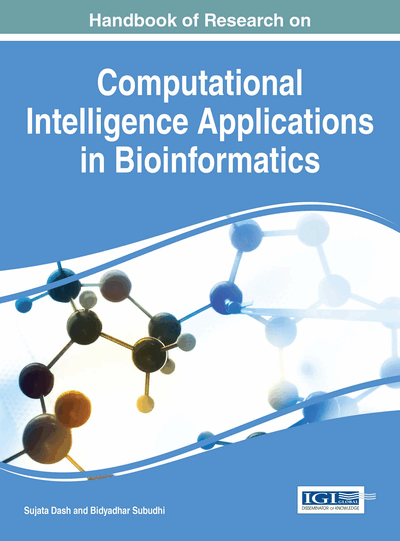# Knowledge Representation: A Semantic Network Approach

Atta ur Rahman (University of Dammam, Saudi Arabia)
DOI: 10.4018/978-1-5225-0427-6.ch004

## Abstract

Knowledge Representation (KR) is an emerging field of research in AI and Data Mining. Knowledge represented in an effective way guarantees a good retrieval. In this regard, a number of effective approaches have been proposed in the literature and Semantic Networks (SN) are one of them. In SN knowledge is represented in the form of directed graph, where concepts and relationships are appeared at vertices and edges respectively. ‘is a' is one of the most frequently used relationship in SN. ‘is a' expresses the exact relationship between any pair of objects. But there exists a huge amount of knowledge that cannot be represented by just ‘is a', like the knowledge where approximations are involved. To overcome this issue, fuzzy semantic networks (FSN) are proposed in this chapter. In FSN ‘is a' is replaced by a fuzzy membership function ‘µ' having value between [0,1]. So the relationship between a pair of concepts can be expressed as a certain degree of membership. This chapter focuses on applications of FSN and its significance over the traditional SN.
Chapter Preview
Top

## Introduction

Knowledge Representation (KR) is one of the hottest areas of research in data mining, AI and big data analytics etc. Knowledge represented in an effective way, helps in easy traversal, searching, reasoning, prediction and inferencing. In this regard, a number of approaches, algorithms, techniques and methods have been proposed in the literature and implemented with their own pros and cons.

KR, though not a new concept, however, its usefulness was not felt as necessary as present. KR is becoming a root of almost every Decision Support System (DSS), Expert Systems (ES) and many other intelligent systems. Moreover, it is one of the prominent component of AI paradigm. A number of techniques for KR are proposed in the literature, Semantic Networks are one of the prominent technique among them. Semantic networks were originally proposed by (Quinlin, 1969). In this technique knowledge is represented by directed graph structure, where vertices (nodes) represent the objects (concepts) and the edges represent the relationship between them. ‘is a’ is one of the most useful relationship between any pair of objects. However, it does not cover the range of relationship between ‘is a’ and ‘is not a’. To overcome this limitation, Semantic Fuzzy Networks (SFN) are proposed in this chapter. Also their applications and implementation details are provided.

This chapter is organized as follows:

• Basic concepts and definitions are presented in first section

• Section two contains a brief introduction of semantic networks

• Section three contains the introduction of fuzzy semantic network concept

• Section four contains the applications of fuzzy semantic network in general

• Section five contains the application of fuzzy semantic network in various domains

## Complete Chapter List

Search this Book:
Reset